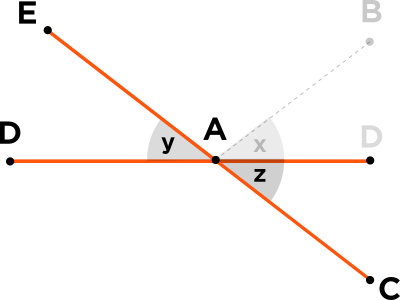# What? Is this an error, or is angle x and y equal?

• Module 2 Week 4 Day 14 Challenge Part 2 Mini-Question

Is this an error, or is angle x and y equal?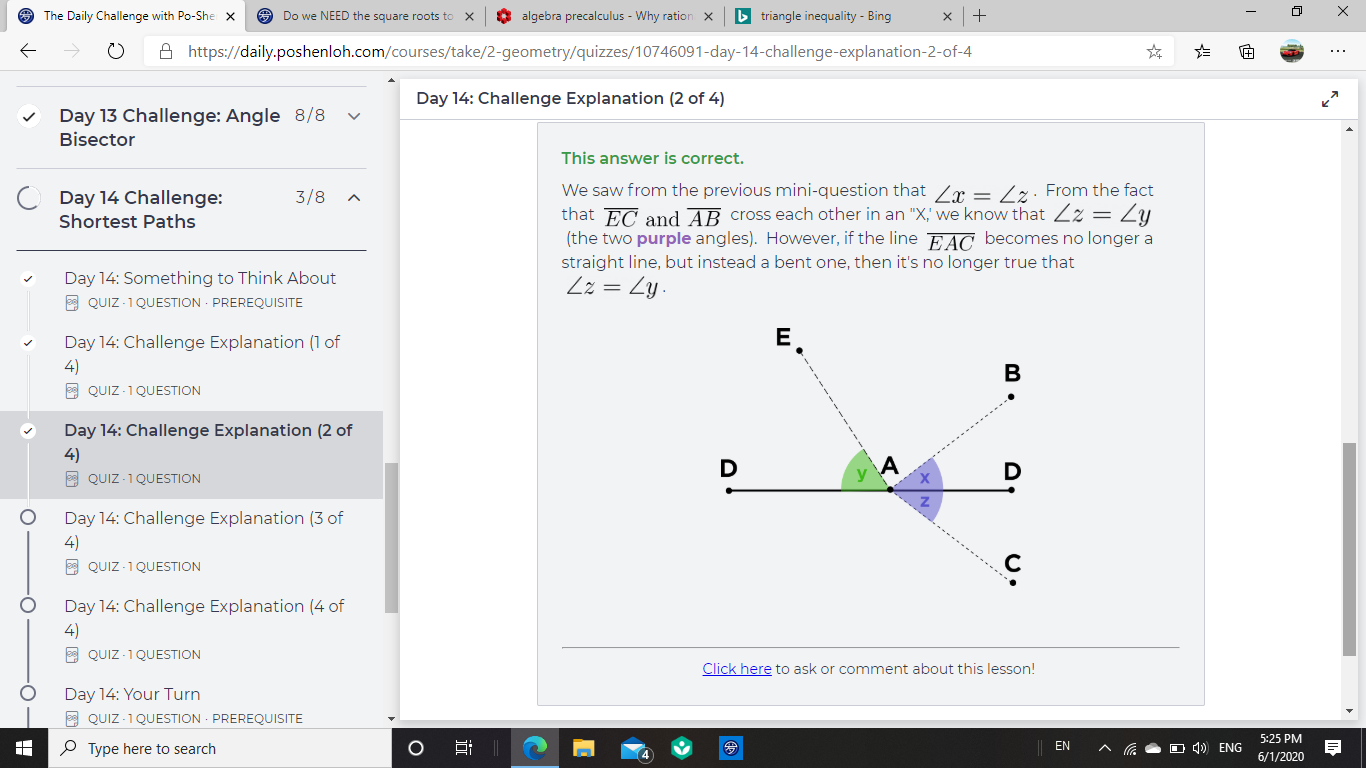• @The-Darkin-Blade This mini-question is trying to ask about what the important relationships in the picture are. In geometry problems, the information we are given is often not written out in sentences or equations, but it's implicitly hidden in the diagram.So, you have to look at the elements of the picture and notice things. This question is trying to help cultivate our ability to sense what the hidden information is, and how to use it effectively.

In the diagram for our Challenge explanation, it indeed looks like $$\angle z = \angle y,$$ and the mini-question starts off with this same picture. In this diagram, $$\angle x = \angle y = \angle z.$$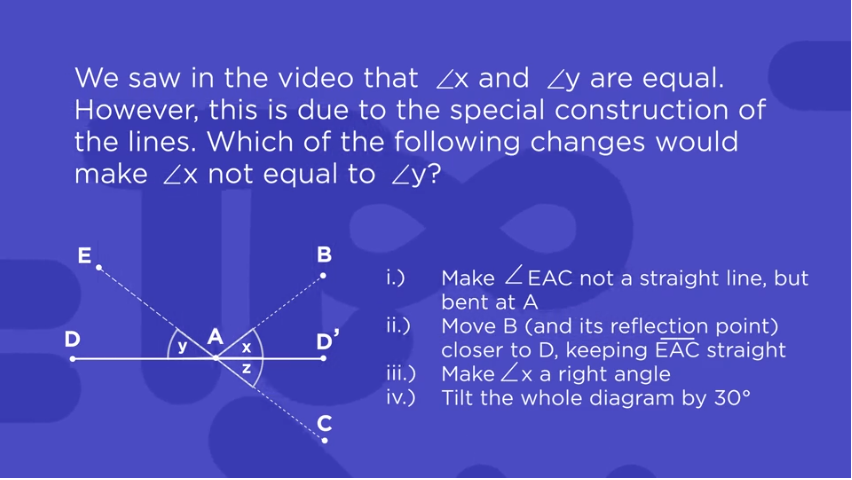This mini-question is asking about what influences the fact that $$\angle x = \angle y = \angle z.$$

The possible answer choices here were:

i.) Make $$\angle EAC$$ not a straight line, but bent at $$A$$
ii.) Move $$B$$ (and its reflection point) closer to $$D,$$ keeping $$\overline{EAC}$$ straight
iii.) Make $$\angle x$$ a right angle
iv.) Tilt the whole diagram by $$30^{\circ}$$

Which of these choices has an effect on whether or not we still $$\angle x = \angle y = \angle z?$$

For choice ii.) if we move $$B$$ closer to $$D,$$ keeping the line $$\overline{EC}$$ straight, we will still have that $$\angle x = \angle y = \angle z.$$ The measure of each angle gets smaller, but they are still all equal to each other.For choice iii.), if we make $$\angle x$$ a right angle, then $$\overline{AB}$$ will be perpendicular ($$\perp$$) to line $$\overline{AD}.$$ Point $$E$$ would coincide at the same place as point $$B.$$ Then, every angle will be a right angle, so $$\angle x = \angle y = \angle z = 90^{\circ}.$$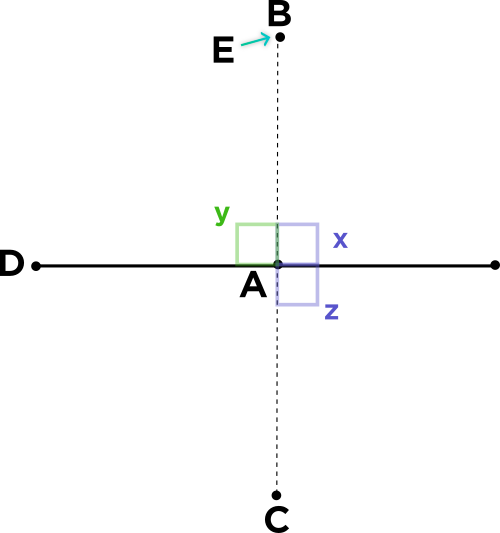For choice iv.), if we tilt the whole diagram, this shouldn't change the relationship of the angles. All the angles would be exactly the same size.

&nbsp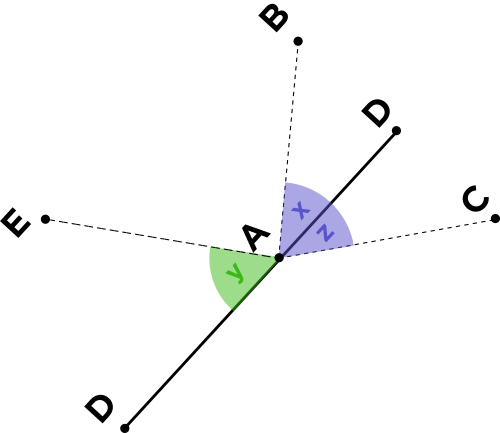If we follow choice i.) and make $$\angle EAC$$ a bent line at point $$A,$$ then we get a diagram that looks like the one below. As you can see, we have found a change to the diagram that makes $$\angle y \neq \angle z!$$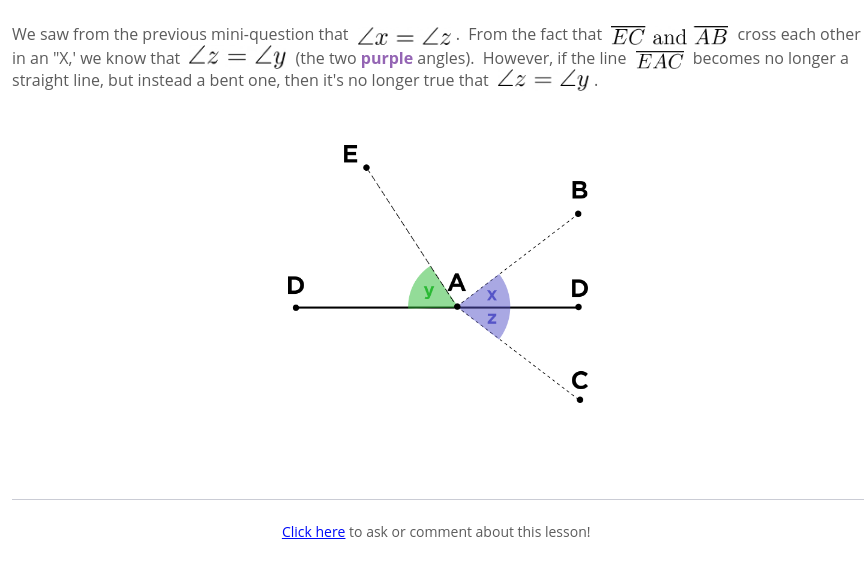By making line $$EC$$ no longer a straight line, we have removed the "X" between lines $$D$$ and $$\overline{EC},$$ which is the reason for $$\angle y = \angle z$$ from before.

In particular, the "X" formed by the two $$\textcolor{red}{\text{red}}$$ lines is what makes it true that $$\angle y = \angle z.$$